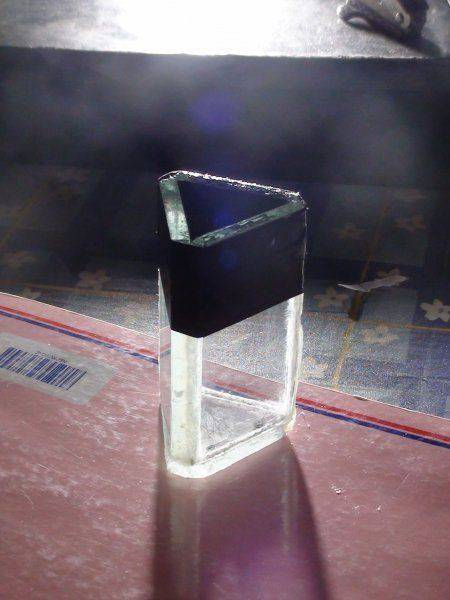# Hollow glass prism

i have recently made a hollow prism for my science exhibition in my school ... to find refractive indices of fluids.

i made it using 3 rectangular pieces of glass (0.5cm) on a glass triangular base ..with obviously a hollow part in the middle
it worked perfectly fine with water i got n=1.33
then my sir came ahead and asked me a question ... how do you find the error in the angle of refraction due to using the thick glass ... i didnt know ..
this is where i reached .. glass is amorphous
so i measured the angle of incidence to be 90 degrees and then i measured the angle of refraction(without anything in the middle of the prism)
i found the difference (which is 1 degree) and multiplied by 2 (as i just found error of 1 glass.)
is my theory correct?

mfb
Mentor
This looks like the measurement uncertainty for angles. The glass will influence the measurement if it does not have the same thickness everywhere, but with an angle of incidence of 90 degrees I don't see how you want to see that.

What did you get as refractive index for air?

i think i got my answer my theory was just wrong ...
the

@mfb
This looks like the measurement uncertainty for angles. The glass will influence the measurement if it does not have the same thickness everywhere, but with an angle of incidence of 90 degrees I don't see how you want to see that.

What did you get as refractive index for air?

i know where i went wrong ... actually you cant find the error of the hollow prism .. it is just like you have a very small glass prism in a water/liquid prism .. the error in the measurement of r will be different for each case

sophiecentaur
Gold Member
2020 Award
IF the glass sides have flat, parallel sides, how much would you expect the angle to be changed by passing through them, with air on both sides? Apply Snell's law at each surface. It would be very easy to calculate the result for a small angle between the faces - Snell's law again. What error can you permit in your overall measurements?

it would differ for different angle of incidences ... because it is a sine proportion ...

sophiecentaur
Gold Member
2020 Award
it would differ for different angle of incidences ... because it is a sine proportion ...
Why, if the faces are parallel? Angle r for the first face is the same as angle i for the second face.
Why would this not apply?

okay guys ... i figured it out
i think there will be no error ..
thank you sophiecentaur
it is because the angle of incidence will be the same as angle of refraction on face 1 as well as face 2 .
there will be lateral deviation but we dont actually need that ...
our aim is the angle of incidence and the angle of emergence and we get that ...
this is the pic of my prism if you want to see itsophiecentaur
Gold Member
2020 Award
You should find this an interesting exercise. Of course, the method is full of possible imperfections and sources of error but you should get a good ball park figure for water and some other liquids. It could be interesting to see how the result changes when the path through the prism is symmetrical and when it's not. How do you plan to plot the ray paths?

we have a soft wood board and pins .. the classic good ol' lab ways.
thanks how do i close this thread?

sophiecentaur